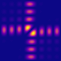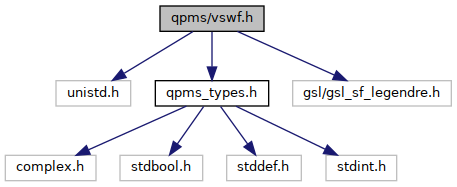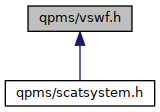QPMS Electromagnetic multiple scattering library and toolkit.
vswf.h File Reference

Vector spherical wavefunctions. More...

#include <unistd.h>
#include "qpms_types.h"
#include <gsl/gsl_sf_legendre.h>
Include dependency graph for vswf.h:This graph shows which files directly or indirectly include this file:Go to the source code of this file.

## Data Structures

struct  qpms_incfield_planewave_params_t
Parameter structure for qpms_incfield_planewave() More...

struct  qpms_vswfset_sph_t
Set of electric and magnetic VSWF values in spherical coordinate basis. More...

## Typedefs

typedef qpms_errno_t(* qpms_incfield_t) (complex double *target, const qpms_vswf_set_spec_t *bspec, const cart3_t evalpoint, const void *args, bool add)
Calculates the (regular VSWF) expansion coefficients of an external incident field. More...

typedef struct qpms_incfield_planewave_params_t qpms_incfield_planewave_params_t
Parameter structure for qpms_incfield_planewave()

typedef struct qpms_vswfset_sph_t qpms_vswfset_sph_t
Set of electric and magnetic VSWF values in spherical coordinate basis. More...

## Functions

qpms_vswf_set_spec_tqpms_vswf_set_spec_init (void)
Creates a qpms_vswf_set_spec_t structure with an empty list of wave indices.

qpms_errno_t qpms_vswf_set_spec_append (qpms_vswf_set_spec_t *self, qpms_uvswfi_t u)
Appends a VSWF index to a qpms_vswf_set_spec_t, also updating metadata.

void qpms_vswf_set_spec_free (qpms_vswf_set_spec_t *)
Destroys a qpms_vswf_set_spec_t.

bool qpms_vswf_set_spec_isidentical (const qpms_vswf_set_spec_t *a, const qpms_vswf_set_spec_t *b)
Compares two vswf basis specs. More...

qpms_vswf_set_spec_tqpms_vswf_set_spec_copy (const qpms_vswf_set_spec_t *orig)
Copies an instance of qpms_vswf_set_spec_t.

qpms_vswf_set_spec_tqpms_vswf_set_spec_from_lMax (qpms_l_t lMax, qpms_normalisation_t norm)
Creates an instance of qpms_vswf_set_spec_t in the 'traditional' layout.

static ssize_t qpms_vswf_set_spec_find_uvswfi (const qpms_vswf_set_spec_t *bspec, const qpms_uvswfi_t index)
Finds the position of a given index in the bspec's ilist. More...

size_t * qpms_vswf_set_reindex (const qpms_vswf_set_spec_t *small, const qpms_vswf_set_spec_t *big)
Creates an index mapping between two bspecs. More...

qpms_errno_t qpms_uvswf_fill (csphvec_t *const target, const qpms_vswf_set_spec_t *setspec, csph_t kr, qpms_bessel_t btyp)
Evaluates a set of VSWF basis functions at a given point. More...

csphvec_t qpms_eval_uvswf (const qpms_vswf_set_spec_t *setspec, const complex double *coeffs, csph_t kr, qpms_bessel_t btyp)
Evaluates field specified by SVWF coefficients at a given point. More...

qpms_errno_t qpms_incfield_planewave (complex double *target, const qpms_vswf_set_spec_t *bspec, const cart3_t evalpoint, const void *args, bool add)
Calculates the (regular VSWF) expansion coefficients of a plane wave. More...

csphvec_t qpms_vswf_single_el (qpms_m_t m, qpms_l_t n, sph_t kdlj, qpms_bessel_t btyp, qpms_normalisation_t norm)
Electric wave N.

csphvec_t qpms_vswf_single_mg (qpms_m_t m, qpms_l_t n, sph_t kdlj, qpms_bessel_t btyp, qpms_normalisation_t norm)
Magnetic wave M.

csphvec_t qpms_vswf_single_el_csph (qpms_m_t m, qpms_l_t n, csph_t kdlj, qpms_bessel_t btyp, qpms_normalisation_t norm)
Electric wave N, complex wave number version.

csphvec_t qpms_vswf_single_mg_csph (qpms_m_t m, qpms_l_t n, csph_t kdlj, qpms_bessel_t btyp, qpms_normalisation_t norm)
Magnetic wave M, complex wave number version..

qpms_errno_t qpms_legendre_deriv_y_get (double **result, double **result_deriv, double x, qpms_l_t lMax, gsl_sf_legendre_t lnorm, double csphase)

qpms_errno_t qpms_legendre_deriv_y_fill (double *where, double *where_deriv, double x, qpms_l_t lMax, gsl_sf_legendre_t lnorm, double csphase)

csphvec_t qpms_vswf_L00 (csph_t kdrj, qpms_bessel_t btyp, qpms_normalisation_t norm)
Evaluate the zeroth-degree longitudinal VSWF $$\mathbf{L}_0^0$$. More...

qpms_errno_t qpms_vswf_fill (csphvec_t *resultL, csphvec_t *resultM, csphvec_t *resultN, qpms_l_t lMax, sph_t kdrj, qpms_bessel_t btyp, qpms_normalisation_t norm)
Evaluate VSWFs at a given point from l = 1 up to a given degree lMax. More...

qpms_errno_t qpms_vswf_fill_alternative (csphvec_t *resultL, csphvec_t *resultM, csphvec_t *resultN, qpms_l_t lMax, sph_t kdrj, qpms_bessel_t btyp, qpms_normalisation_t norm)

qpms_errno_t qpms_vswf_fill_csph (csphvec_t *resultL, csphvec_t *resultM, csphvec_t *resultN, qpms_l_t lMax, csph_t kdrj, qpms_bessel_t btyp, qpms_normalisation_t norm)
Evaluate VSWFs at a given point from l = 1 up to a given degree lMax (complex kr version). More...

qpms_errno_t qpms_vecspharm_fill (csphvec_t *const a1target, csphvec_t *const a2target, csphvec_t *const a3target, qpms_l_t lMax, sph_t dir, qpms_normalisation_t norm)
Evaluate vector spherical harmonics at dir. More...

qpms_errno_t qpms_vecspharm_dual_fill (csphvec_t *const a1target, csphvec_t *const a2target, csphvec_t *const a3target, qpms_l_t lMax, sph_t dir, qpms_normalisation_t norm)

qpms_errno_t qpms_planewave2vswf_fill_cart (cart3_t wavedir, ccart3_t amplitude, complex double *targt_longcoeff, complex double *target_mgcoeff, complex double *target_elcoeff, qpms_l_t lMax, qpms_normalisation_t norm)

qpms_errno_t qpms_planewave2vswf_fill_sph (sph_t wavedir, csphvec_t amplitude, complex double *targt_longcoeff, complex double *target_mgcoeff, complex double *target_elcoeff, qpms_l_t lMax, qpms_normalisation_t norm)

csphvec_t qpms_eval_vswf (sph_t where, complex double *longcoeffs, complex double *mgcoeffs, complex double *elcoeffs, qpms_l_t lMax, qpms_bessel_t btyp, qpms_normalisation_t norm)

csphvec_t qpms_eval_vswf_csph (csph_t where, complex double *longcoeffs, complex double *mgcoeffs, complex double *elcoeffs, qpms_l_t lMax, qpms_bessel_t btyp, qpms_normalisation_t norm)

qpms_vswfset_sph_tqpms_vswfset_make (qpms_l_t lMax, sph_t kdlj, qpms_bessel_t btyp, qpms_normalisation_t norm)

void qpms_vswfset_sph_pfree (qpms_vswfset_sph_t *)

## Detailed Description

Vector spherical wavefunctions.

N.B. for the Legendre polynomial norm definitions, see the corresponding section of GSL docs or gsl/specfunc/legendre_source.c.

## ◆ qpms_incfield_t

 typedef qpms_errno_t(* qpms_incfield_t) ( complex double *target, const qpms_vswf_set_spec_t *bspec, const cart3_t evalpoint, const void *args, bool add)

Calculates the (regular VSWF) expansion coefficients of an external incident field.

Parameters
 target Target non-NULL array of the regular VSWF expansion coefficients of length bspec->n. evalpoint Point at which the VSWF expansion is made. args Pointer to additional function-specific arguments. add If true, add to target; rewrite target if false.

## ◆ qpms_vswfset_sph_t

 typedef struct qpms_vswfset_sph_t qpms_vswfset_sph_t

Set of electric and magnetic VSWF values in spherical coordinate basis.

This is supposed to contain all the waves up to $l = lMax$.

For a completely custom set of waves, use qpms_uvswfset_sph_t instead.

## ◆ qpms_eval_uvswf()

 csphvec_t qpms_eval_uvswf ( const qpms_vswf_set_spec_t * setspec, const complex double * coeffs, csph_t kr, qpms_bessel_t btyp )

Evaluates field specified by SVWF coefficients at a given point.

SVWF coefficients in coeffs must be ordered according to setspec->ilist.

Parameters
 coeffs SVWF coefficient vector of size setspec->n. kr Evaluation point.

## ◆ qpms_incfield_planewave()

 qpms_errno_t qpms_incfield_planewave ( complex double * target, const qpms_vswf_set_spec_t * bspec, const cart3_t evalpoint, const void * args, bool add )

Calculates the (regular VSWF) expansion coefficients of a plane wave.

The wave amplitude and wave vector is defined by struct qpms_incfield_planewave_params_t.

If the wave vector and amplitude are not orthogonal (i.e. the plane wave is not fully transversal) and bspec->lMaxL is non-negative, the corresponding longitudinal components are calculated as well.

For complex k vectors, the implementation is not completely correct right now. Locally, it corresponds to decomposition of a plane wave with a real k (using the real part of the k supplied), just the whole decomposition is modulated by the origin-dependent factor $$\vect E e^{i \vect k \cdot \vect r}$$ with $$\vect k$$ complex.

Parameters
 target Target non-NULL array of the regular VSWF expansion coefficients of length bspec->n. evalpoint Point at which the VSWF expansion is made. args Pointer to additional function-specific arguments (converted to (const qpms_incfield_planewave_params_t *)). add If true, add to target; rewrite target if false.

## ◆ qpms_uvswf_fill()

 qpms_errno_t qpms_uvswf_fill ( csphvec_t *const target, const qpms_vswf_set_spec_t * setspec, csph_t kr, qpms_bessel_t btyp )

Evaluates a set of VSWF basis functions at a given point.

The list of basis wave indices is specified in setspec; setspec->norm must be set as well.

Parameters
 target Target array of size at least setspec->n. kr Evaluation point.

## ◆ qpms_vecspharm_fill()

 qpms_errno_t qpms_vecspharm_fill ( csphvec_t *const a1target, csphvec_t *const a2target, csphvec_t *const a3target, qpms_l_t lMax, sph_t dir, qpms_normalisation_t norm )

Evaluate vector spherical harmonics at dir.

The length of each of the target arrays shall be lMax * (lMax + 2). If any of the target arrays pointers is NULL, the corresponding VSH will not be evaluated. The "zeroth" radial VSH $$\vshrad_0^0$$ is not evaluated.

Parameters
 a1target Target array for radial VSH $$\vshrad$$. a2target Target array for "rotational" VSH $$\vshrot$$. a3target Target array for "gradiental" VSH $$\vshgrad$$.

## ◆ qpms_vswf_fill()

 qpms_errno_t qpms_vswf_fill ( csphvec_t * resultL, csphvec_t * resultM, csphvec_t * resultN, qpms_l_t lMax, sph_t kdrj, qpms_bessel_t btyp, qpms_normalisation_t norm )

Evaluate VSWFs at a given point from l = 1 up to a given degree lMax.

The target arrays resultL, resultM, resultN have to be large enough to contain lMax * (lMax + 2) elements. If NULL is passed instead, the corresponding SVWF type is not evaluated.

Does not evaluate the zeroth-order wave $$\mathbf{L}_0^0$$. If you need that, use qpms_vswf_L00().

If kdrj.r == 0 and btyp != QPMS_BESSEL_REGULAR, returns QPMS_ESING and fills targets with NaNs.

Returns
0 (QPMS_SUCCESS) on success, otherwise.

## ◆ qpms_vswf_fill_csph()

 qpms_errno_t qpms_vswf_fill_csph ( csphvec_t * resultL, csphvec_t * resultM, csphvec_t * resultN, qpms_l_t lMax, csph_t kdrj, qpms_bessel_t btyp, qpms_normalisation_t norm )

Evaluate VSWFs at a given point from l = 1 up to a given degree lMax (complex kr version).

The target arrays resultL, resultM, resultN have to be large enough to contain lMax * (lMax + 2) elements. If NULL is passed instead, the corresponding SVWF type is not evaluated.

Does not evaluate the zeroth-order wave $$\mathbf{L}_0^0$$. If you need that, use qpms_vswf_L00().

## ◆ qpms_vswf_L00()

 csphvec_t qpms_vswf_L00 ( csph_t kdrj, qpms_bessel_t btyp, qpms_normalisation_t norm )

Evaluate the zeroth-degree longitudinal VSWF $$\mathbf{L}_0^0$$.

Any norm is being ignored right now.

## ◆ qpms_vswf_set_reindex()

 size_t* qpms_vswf_set_reindex ( const qpms_vswf_set_spec_t * small, const qpms_vswf_set_spec_t * big )

Creates an index mapping between two bspecs.

Creates an array r such that small->ilist[i] == big->ilist[r[i]]. It's not lossless if the two bspecs contain different combinations of waves.

Preferably, big->ilist contains everything small->ilist does. If small->ilist[i] is not found in big->ilist, r[i] will be set to ~(size_t)0.

## ◆ qpms_vswf_set_spec_find_uvswfi()

 static ssize_t qpms_vswf_set_spec_find_uvswfi ( const qpms_vswf_set_spec_t * bspec, const qpms_uvswfi_t index )
inlinestatic

Finds the position of a given index in the bspec's ilist.